Algebra 1 5-1 Complete Lesson: Rate of Change and Slope
starstarstarstarstarstarstarstarstarstar
5 (2 ratings)
by Matthew Richardson
| 31 Questions
Note from the author:
A complete formative lesson with embedded slideshow, mini lecture screencasts, checks for understanding, practice items, mixed review, and reflection. I create these assignments to supplement each lesson of Pearson's Common Core Edition Algebra 1, Algebra 2, and Geometry courses. See also mathquest.net and twitter.com/mathquestEDU.The outlined content above was added from outside of Formative.1
2
1
10 pts
Solve It! The table shows the horizontal and vertical distances from the base of the mountain at several poles along the path of a ski lift. The poles are connected by a cable. Between which two poles is the cable's path the steepest?
Pole A
Pole B
Pole C
Pole D
2
10 pts
Solve It! How do you know?3
3
5 pts
Problem 1 Got It?
A
B4
4
10 pts
Problem 2 Got It?
A
B
C
D5
5
10 pts
Problem 2 Got It?
A
B
C
D6
6
5 pts
Problem 2 Got It?
A
B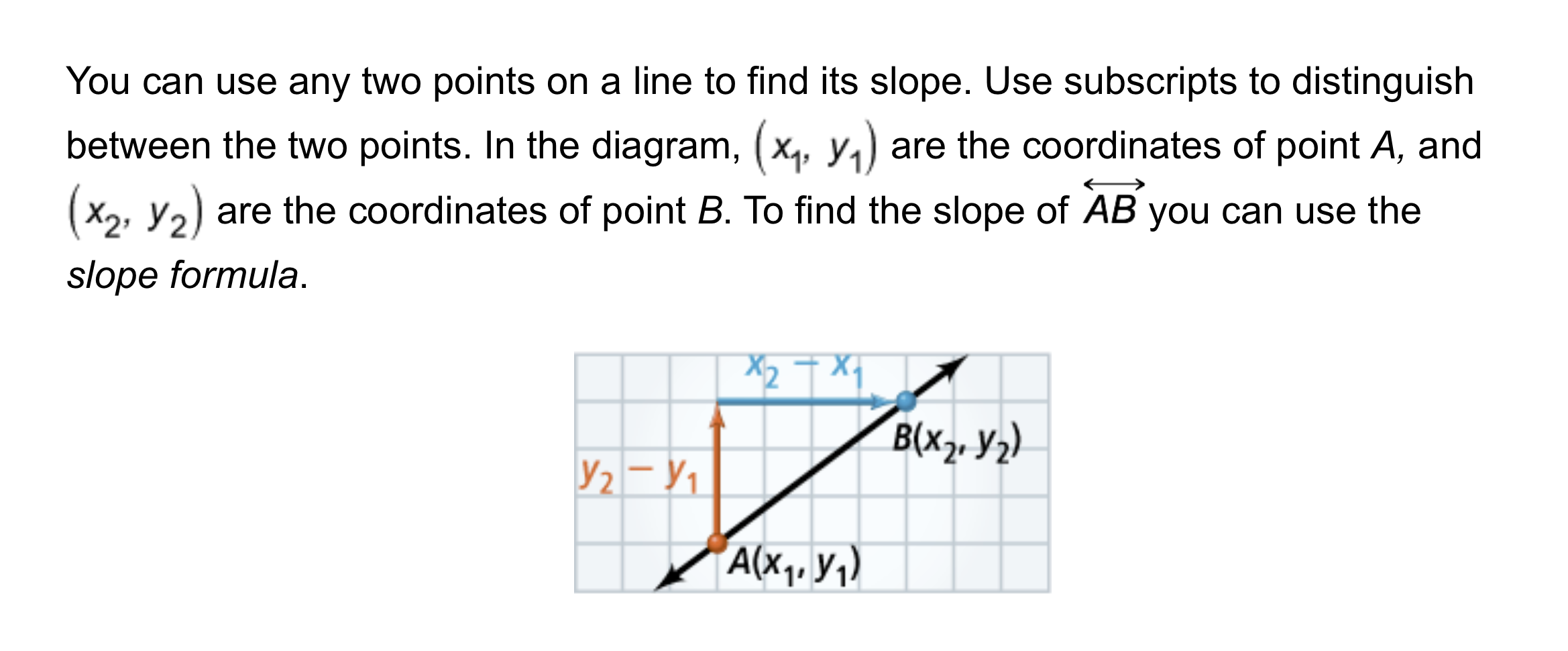7
10 pts
Problem 3 Got It? What is the slope of the line through the points (1, 3) and (4, -1)?
Enter only a number in fraction form.
8
10 pts
Problem 3 Got It? Reasoning: Plot the points (1, 3) and (4, -1) from the previous item and draw a line through them. Include relevant graph detail: label axes, indicate scale on both axes, add arrows to the ends of lines and axes.
9
10 pts
Problem 3 Got It? Reasoning: Does the slope of the line you drew in the previous item match the slope you calculated in the item before that? Explain.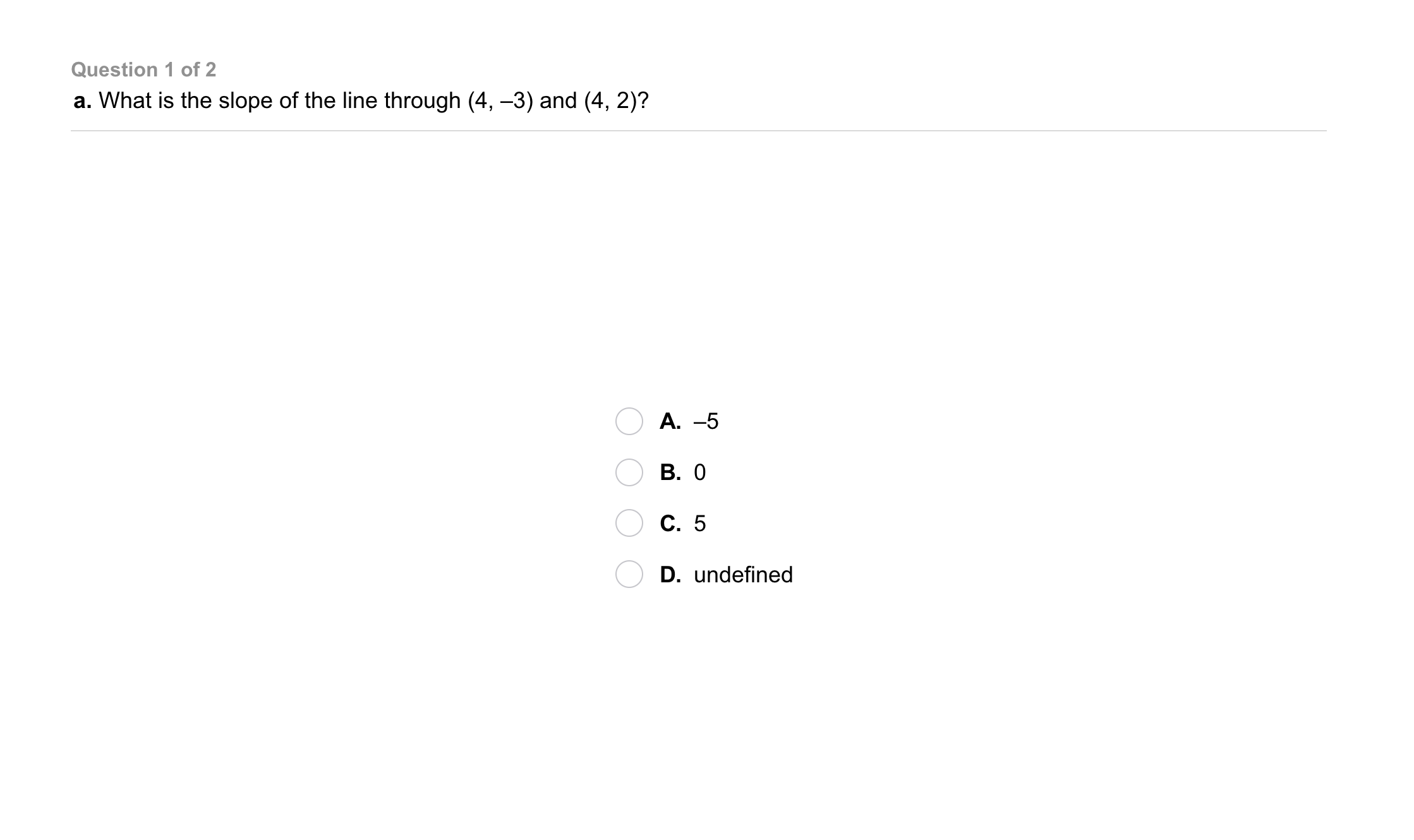10
10
10 pts
Problem 4 Got It?
A
B
C
D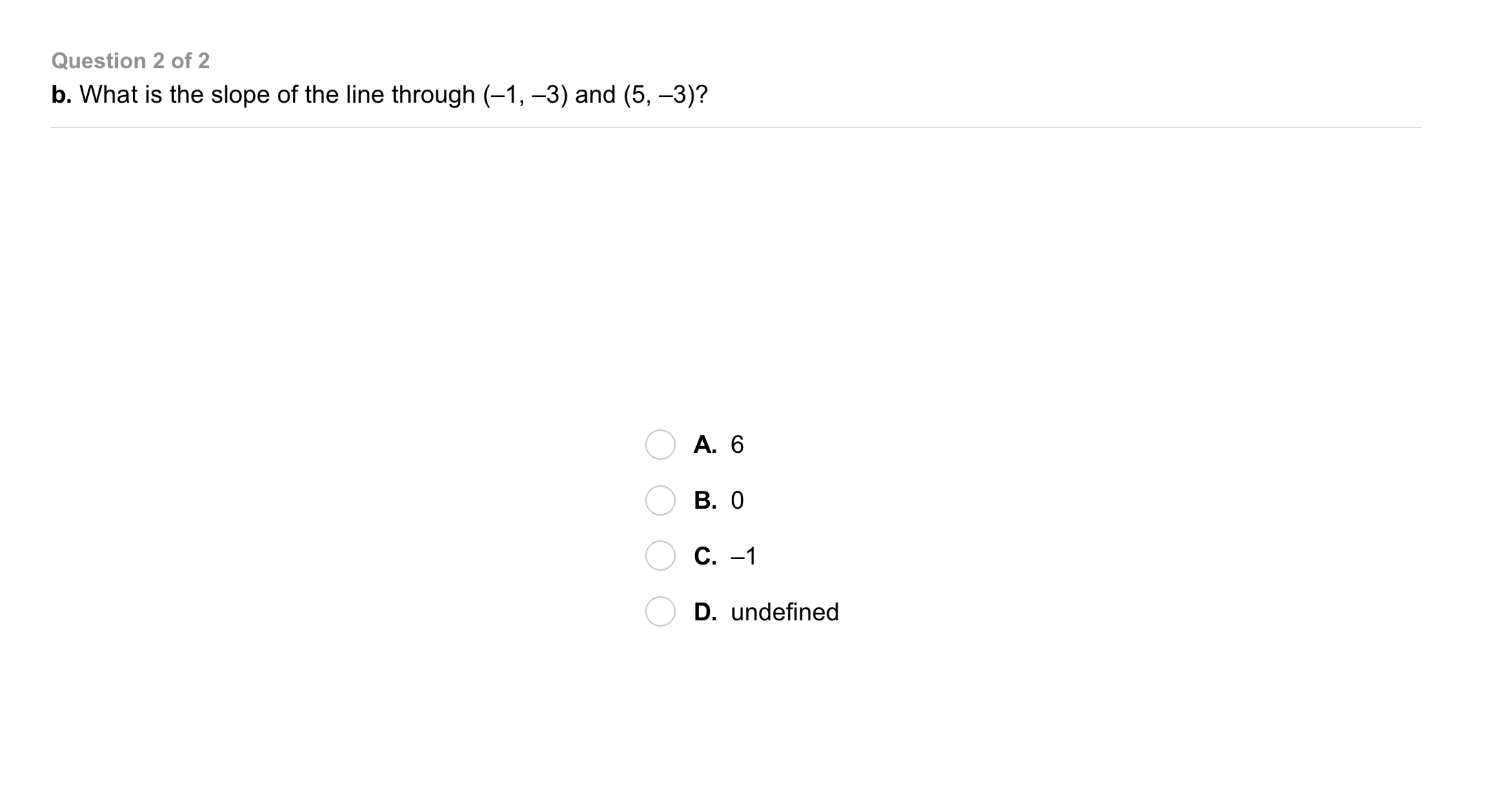11
11
10 pts
Problem 4 Got It?
A
B
C
D12
12
5 pts
A
B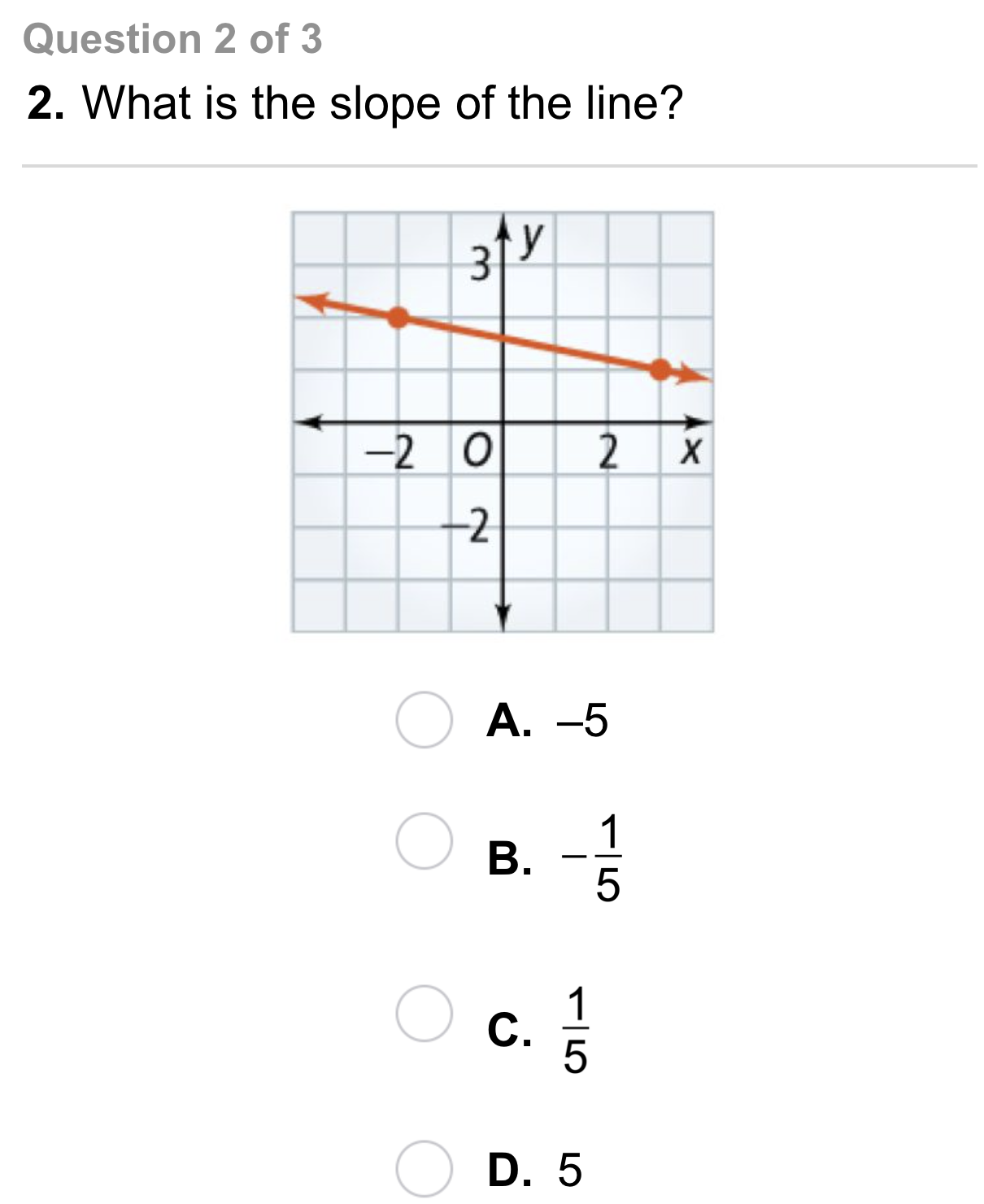13
13
10 pts
A
B
C
D14
14
10 pts
A
B
C
D15
10 pts
Vocabulary: What characteristic of a graph represents rate of change?
The x-intercept
The slope
The y-intercept
16
10 pts
Open-Ended: Give an example of a real-world situation that you can model with a horizontal line. What is the rate of change for the situation? Explain.17
18
17
10 pts
Error Analysis: A student calculated the slope of the line shown to be 2. What is the correct slope?
2
-2
1/2
-1/2
18
10 pts
Error Analysis: Explain the student's mistake.19
10 pts
Review Lesson 4-7: Identify the second, fourth, and tenth terms of the sequence.

5
7
9
19
21
20
10 pts
Review Lesson 4-7: Identify the second, fourth, and tenth terms of the sequence.

-5
0
1
7
13
49
21
10 pts
Review Lesson 3-8: Use the sets below to build each union or intersection on the right of elements on the left.

• 1
• 2
• 3
• 4
• 5
• 6
• 7
• 8
• 9
• 10
• { }
• A ∩ B
• A ∩ C
• B ⋃ C
22
10 pts
Review Lesson 2-7: Solve the proportion.
13
3.3
6.8
7.5
23
10 pts
Review Lesson 2-7: Solve the proportion.
Enter only a number.
24
10 pts
Review Lesson 2-7: Solve the proportion.
Enter only a number.
25
10 pts
Review Lesson 2-7: Solve the proportion.
Enter only a number.26
10 pts
Vocabulary Review: Use the terms on the left to complete the sentences on the right.
• always
• sometimes
• never
• division
• multiplication
• different
• the same
• A rate is __?__ a ratio.
• A ratio is __?__ a rate.
• A rate compares two quantities by __?__.
• A rate compares quantities in __?__ unit(s).27
10 pts
Use Your Vocabulary: How does the slope of a road affect a person's driving?
28
10 pts
Use Your Vocabulary: What kind of ski slope would a beginning skier use.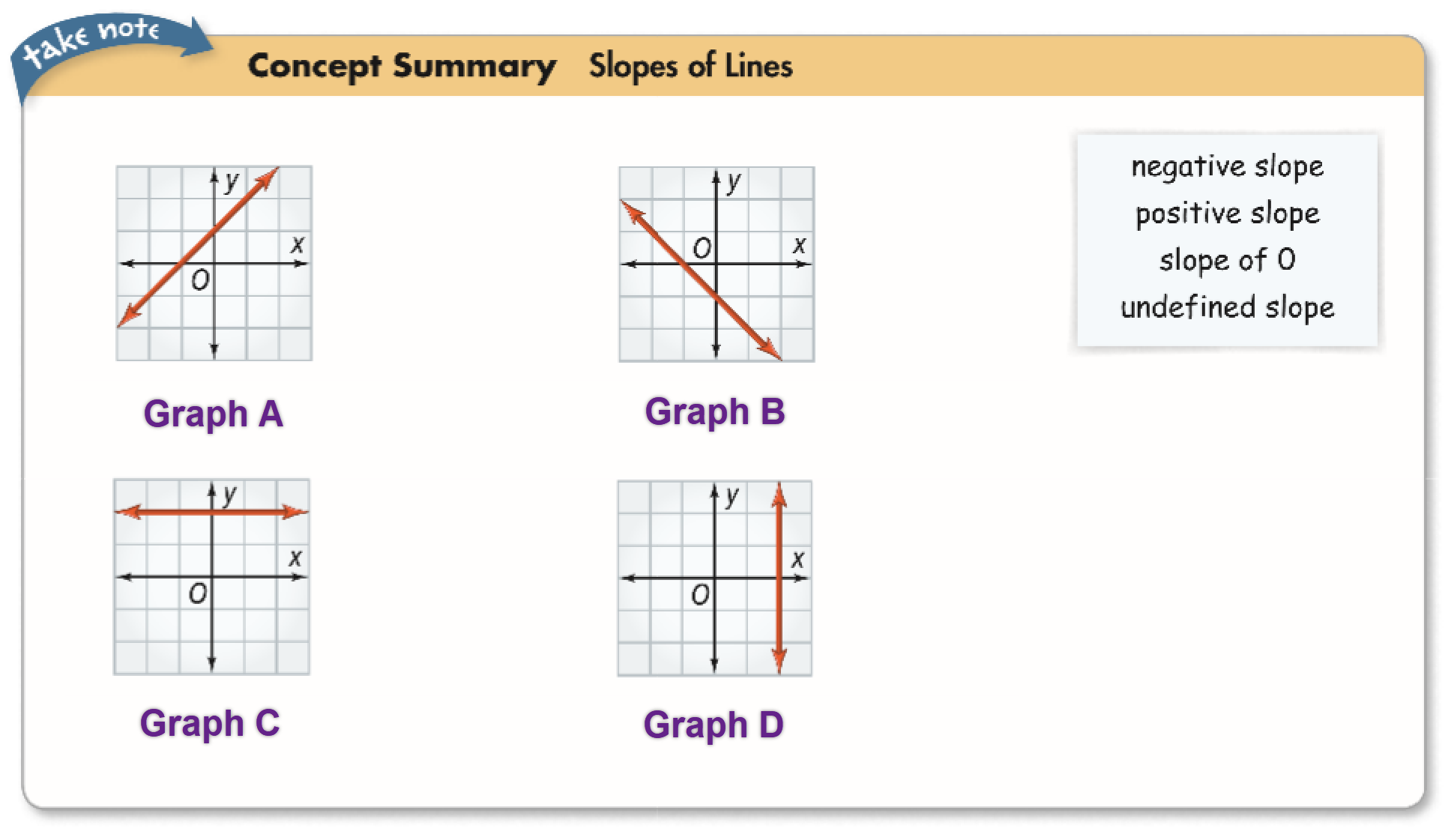29
29
10 pts
Concept Summary: Slopes of Lines Identify the type of slope shown by the line in each graph.
• negative slope
• positive slope
• slope of 0
• undefined slope
• Graph A
• Graph B
• Graph C
• Graph D
30
100 pts
Notes: Take a clear picture or screenshot of your Cornell notes for this lesson. Upload it to the canvas. Zoom and pan as needed.

For a refresher on the Cornell note-taking system, click here.
31
10 pts
Reflection: Math Success
Add to my formatives list

Formative uses cookies to allow us to better understand how the site is used. By continuing to use this site, you consent to the Terms of Service and Privacy Policy.